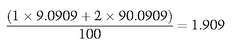TECHNICAL APPENDICES

# APPENDIX A DURATION

An important concept in the bond markets is that of duration. We can define duration as the weighted average time until the receipt of cash flows from an instrument, where the weights are the present values of the cash flows. More formally we can write:
(A.1)where
D = Duration;
P = Price of the bond;
Ct = Cash flow at time t;
r = Yield.
In the case of a zero-coupon bond there is only one cash flow, the payment at maturity. Therefore, for zero-coupon bonds the duration is always equal to the maturity of the bond. Consider now a 10% 2-year bond yielding 10% today: the cash flows are £10 in 1 year’s time and £110 in 2 years′ time. Viewed as two zero-coupon bonds the cash flows would now be a 1-year zero of £10 and a 2-year zero of £110. The duration of the combined package will be the average duration of the two bonds weighted by the size of the bond. Since we are measuring the duration today it is sensible to weight the two bonds in terms of today’s money in present value terms. Since the bond is a 10% bond and we are valuing it at a yield of 10% the price is par, or 100. So the duration calculation is:This incidentally illustrates the point that for a coupon-bearing bond the duration is always less than for the corresponding zero-coupon ...

Get An Introduction to Repo Markets, Third Edition now with the O’Reilly learning platform.

O’Reilly members experience live online training, plus books, videos, and digital content from nearly 200 publishers.